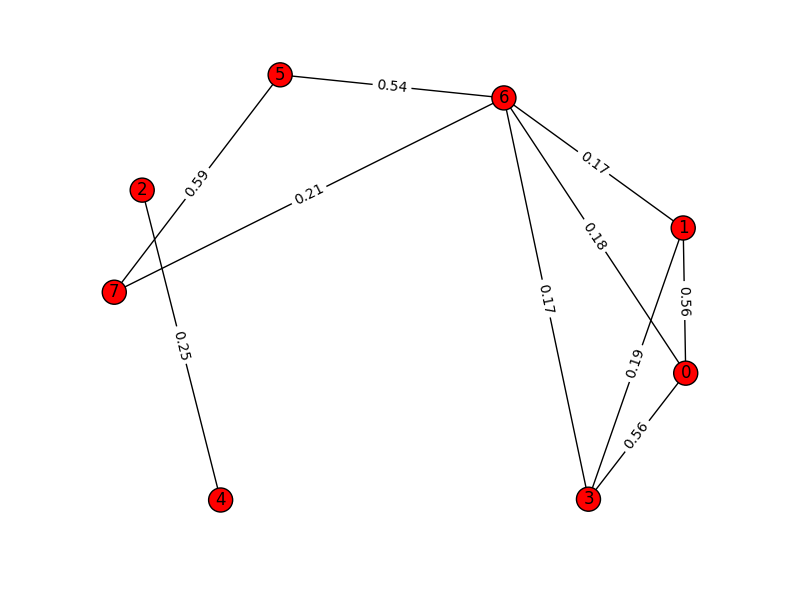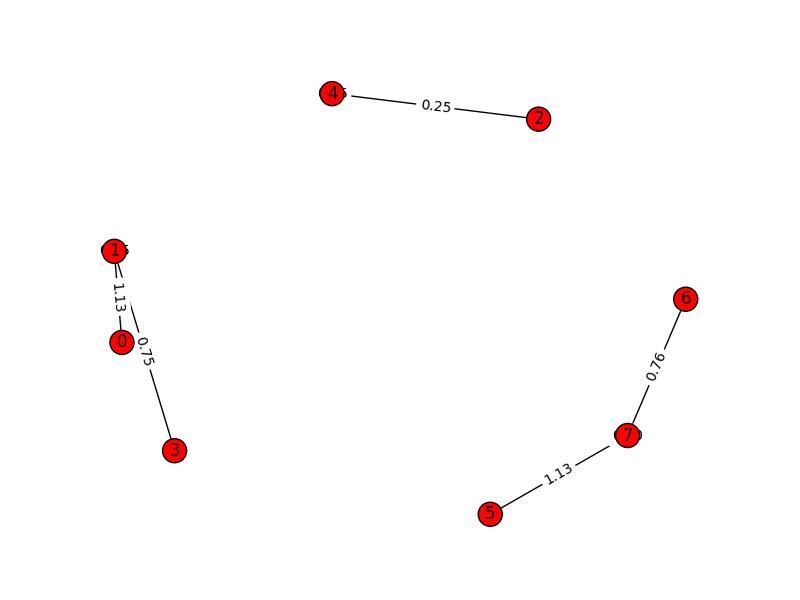# Clustering text using MajorClust

TL;DR here is public gist

## Introduction

I had a conversation with 9 about clustering links based on content and he pointed me to a StackOverflow(SO) conversation about getting started with it. It is very simple concept, but I had to stare at the pseudo code of paper, StackOverflow, results of different sample-sets, for quite some time to understand what is actually happening.

```n = 0, t = false
v   V do n = n + 1, c(v) = n end
while t = false do
t = true
v   V do
c  = i if  (u, v) is max.
if c(v) != c  then c(v) = c  , t = false
end
end
```

For example dataset mentioned in StackOverflow

```texts = [
"foo blub baz",
"foo bar baz",
"asdf bsdf csdf",
"foo bab blub",
"csdf hddf kjtz",
"123 456 890",
"321 890 456 foo",
"123 890 uiop",
]
```

If we try to plot initial graph based on cosine distance among all the nodes(each element of texts list) we get something like this:And once we cluster the graph using MajorClust algorithm, the graph get restructured to:Notice how edges get aggregated and new weights are assigned to edges based on clusters and not-so-important edges are dropped altogether.

## Code

The code on StackOverflow thread is complete without any external dependencies. I used TfidfVectorizer from sklearn to create vector space model from the documents(different and bigger dataset).

```# corpus is list of all the documents
vectorizer = TfidfVectorizer(stop_words = "english",
ngram_range = (2, 3),
max_features = 100000)
corpus_mat = vectorizer.fit_transform(corpus)
num_of_samples, num_of_features = corpus_mat.shape
```

Then, I calculate cosine distance of each vector(document) from other vectors.

```magnitudes = np.zeros((num_of_samples))
# this loop can be removed?
for doc_id in range(num_of_samples):
magnitudes[doc_id] = np.sqrt(corpus_mat[doc_id].dot(corpus_mat[doc_id].T).sum())

cosine_distances = np.zeros((num_of_samples, num_of_samples))
# this loop can be improved
for doc_id, other_id in combinations(range(num_of_samples), 2):
distance = (corpus_mat[doc_id].dot(corpus_mat[other_id].T).sum())/(magnitudes[doc_id]*magnitudes[other_id])
cosine_distances[doc_id, other_id] = cosine_distances[other_id, doc_id] = distance
```

This cosinedistances matrix is adjacency matrix representation of the graph, where each node is one document and edge weight(cosine distance) being the measure of similarity among two documents. Now MajorClust algorithm is clustering based on aggregating/accumulating edge weights and assigning each node to cluster which has maximum accumulated edge weight.

```t = False
indices = np.arange(num_of_samples)
while not t:
t = True
for index in np.arange(num_of_samples):
# aggregating edge weights
new_index = np.argmax(np.bincount(indices,
weights=cosine_distances[index]))
if indices[new_index] != indices[index]:
indices[index] = indices[new_index]
t = False

clusters = {}
for item, index in enumerate(indices):